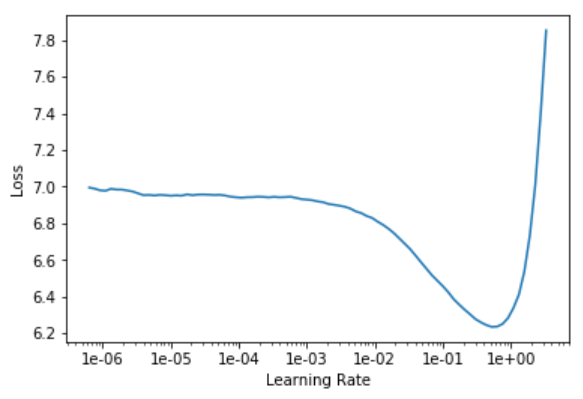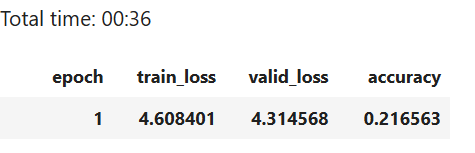# FastAI Sentiment AnalysisSentiment analysis refers to the use of natural language processing, text analysis, computational linguistics, and other techniques to identify and quantify the sentiment (i.e. positive, neutral, or negative) of text or audio data.

Because it’s really hard for a model to learn language when only provided with a single value — the sentiment — FastAI lets you first train a language  model — a model that predicts the next word — and then use that encoder for the model that actually classifies the sentiment.

This allows the classification model  to focus on learning the sentiment instead of having to learn the whole language from scratch. This approach offers astonishingly good results.

For our data, we’ll use the Twitter US Airline Sentiment dataset from Kaggle. The dataset contains tweets about US Airlines, annotated with their respective sentiments.

After downloading the dataset, we need to import the text module from the FastAI library, specify the path to our dataset, and load in our csv using pandas.

from fastai.text import *

# specify path
path = Path('<Path to the data-set>')

print(df.head())

The two columns that are important for our problem are the airline_sentiment column, which contains a sentiment value —if you’ll recall, either negative, neutral, or positive — and the text column.

As mentioned above, we’ll create two models. A language model and a text   classification model, therefore we’ll create two different data bunches using the TextLMDataBunch and TextClasDataBunch classes:

# split df into training and validation
train_df, valid_df = df.loc[:12000,:], df.loc[12000:,:]

# create databunches
data_lm = TextLMDataBunch.from_df(path, train_df, valid_df, text_cols=10, bs=32)
data_clas = TextClasDataBunch.from_df(path, train_df, valid_df, text_cols=10, label_cols=1, bs=32)

We can get a few examples of each data bunch using the show_batch method:

data_lm.show_batch()
data_clas.show_batch()

You  may notice that there are quite a few strange tokens starting with xx. These are special FastAI tokens that have the following meanings:

• xxbos: Beginning of a text.
• xxfld: Represents separate parts of your document (several columns in a dataframe) like headline, body, summary, etc.
• xxmaj: Indicates that the next word starts with a capital, e.g. “House” will be tokenized as “xxmaj house”.
• xxup: Indicates that next word is written in all caps, e.g. “WHY” will be tokenized as “xxup why ”.
• xxrep: Token indicates that a character is repeated n times, e.g. if you have  10 $in a row it will be tokenized as “xxrep 10$” (in general “xxrep n  {char}”)
• xxwrep: Indicates that a word is repeated n times.
• xxpad : Token used as padding (so every text has the same length)

### Language model

In FastAI, a language model can be created using the language_model_learner method. We’ll pass this method three arguments. Our data, a pre-trained model   (trained on Wikipedia text), and a dropout percentage.

After creating the model, we’ll follow the standard FastAI training pipeline, which includes finding the best learning rate, training the top layers, unfreezing all layers, and repeating the above process.

If you aren’t familiar with this process yet, I’d highly recommend checking out the Practical Deep Learning for Coders course, which is excellent for anyone who wants to learn about cutting edge deep learning approaches. Or you can check out my first FastAI tutorial, which goes into this process in detail.

learn = language_model_learner(data_lm, pretrained_model=URLs.WT103, drop_mult=0.3)

learn.lr_find() # find learning rate
learn.recorder.plot() # plot learning rate graphFigure 5: Learning rate helps us find the optimal learning rate as described here.
learn.fit_one_cycle(1, 1e-2)Figure 6: Training results (shows loss and accuracy metrics after the end of the epoch)
learn.unfreeze() # must be done before calling lr_find
learn.lr_find()
learn.recorder.plot()
learn.fit_one_cycle(10, 1e-3)

Now that we have our trained language model, we can make predictions and save the encoder to use for our classification model:

# make predictions
TEXT = "I liked "
N_WORDS = 40
N_SENTENCES = 2
print("\n".join(learn.predict(TEXT, N_WORDS, temperature=0.75) for _ in range(N_SENTENCES)))

# save encoder
learn.save_encoder('twitter-sentiment-enc')

### Classification model

With our language model ready and the encoder saved, we can now create a text classification model, load in the encoder, and train the network.

# create model and load in encoder
learn = text_classifier_learner(data_clas, pretrained_model=URLs.WT103, drop_mult=0.3)

# find and plot learning rate
learn.lr_find()
learn.recorder.plot()

For training the classification model, we’ll start off by training the  decoder, and then we’ll unfreeze one layer after another—this approach was found to give better results for text classification models.

learn.fit_one_cycle(1, 1e-2)

# unfreeze one layer group and train another epoch
learn.freeze_to(-2)
learn.fit_one_cycle(1, slice(5e-3/2., 5e-3))

learn.freeze_to(-3)
learn.fit_one_cycle(1, slice(5e-3/2., 5e-3))

learn.unfreeze()
learn.fit_one_cycle(1, slice(2e-3/100, 2e-3))

With our model trained, we can now make predictions using the predict method:

learn.predict("I really loved the flight")

This outputs a tuple containing the class as a string and integer as well as the probability values for each class.

Out: (Category positive, tensor(2), tensor([0.1067, 0.0125, 0.8808]))

### Conclusion

The FastAI library offers us a high-level API capable of creating deep learning models for a lot of different applications, including text generation, text analysis, image classification, and image segmentation.

For more information on the FastAI library check out the Practical Deep Learning for Coders course, which goes through a lot of cutting edge deep learning techniques.

If you liked this article consider subscribing to my YouTube Channel and following me on social media.